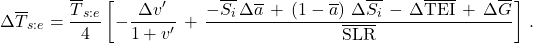# Analysis: Planetary Temperature – Parameterized by Greenhouse Effect Flux Difference

In a previous analysis, I derived a rigorous formula for planetary temperature. That derivation characterized the greenhouse effect using the normalized greenhouse effect parameter,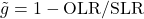, where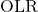is the outgoing longwave radiation flux, and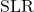is the surface-emitted longwave radiation flux. However,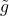is only one possible parameter that can be used to characterize the greenhouse effect. In this analysis, I will offer another (equally rigorous) version of the planetary temperature formula, using an alternate way of expressing the greenhouse effect.

## Greenhouse effect flux difference

The greenhouse effect flux difference is defined as:

(1)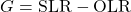so that

(2)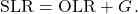## Non-equilibrium temperature formula

From the prior analysis of longwave radiation:

(3)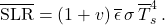The overbar notation is used to denote quantities suitable for using in global time-averaged equations.

From prior analysis, conservation of energy may be expressed as

(4)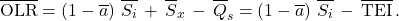where the global TOA energy imbalance,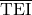, is related to the global energy storage rate,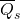, and the non-solar heating rate,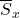, by:

(5)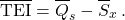Combining the preceding equations allows one to solve for the global mean surface temperature,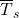:

(6)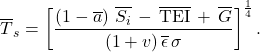The above formula for surface temperature must always be satisfied, whether or not there is equilibrium.

## Equilibrium temperature formula

The energy storage term can be expanded as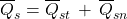where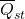is the thermal-energy storage rate and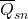is the non-thermal-energy storage rate. Temperature will be stable if the thermal energy storage rate is zero. Hence, the equilibrium temperature is given by:

(7)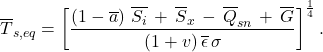## Small changes

To consider the effect of small changes, it is useful to rearrange the formula for conservation of energy (or surface temperature) as follows:

(8)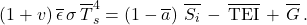A similar equation needs to be satisfied for the variables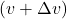,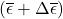,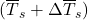,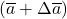,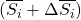,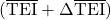, and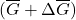. If we expand that similar equation and retain only first-order change terms, and subtract off the above equation, one obtains:

(9)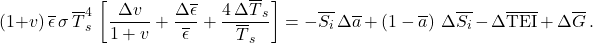Using the prior equation for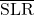and solving for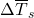leads to:

(10)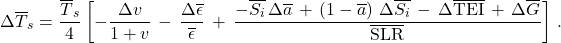## Greenhouse effect forcing

A “forcing” in the greenhouse effect is typically characterized by the change towhen no other parameters are allowed to change. Given the above formula for “small changes”, one can infer that ifchanges by an amount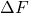, then this will be related to the change in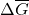by:

(11)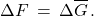## Simple response to forcing

If a forcing results in a change inby an amount, then the temperature subsequently changes to cancel out this imbalance, with no other variables changing, then one finds:

(12)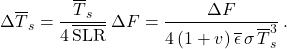## Surface Effective Radiative Emission Temperature

When working with some datasets, it may be convenient to work with the surface “effective radiative emission temperature” (or, more concisely, “effective temperature”) rather than the actual surface temperature. The local surface effectve temperature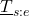is given by:

(13)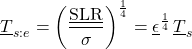The surface effective temperature may be useful to work with because it can be determined by knowing the surface longwave emissions,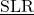, even if the emissivity of the surface is unknown.

The general (non-equilibrium) equation for surface effective temperature is:

(14)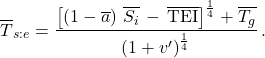The small-changes equation for the surface effective temperature is:

(15)